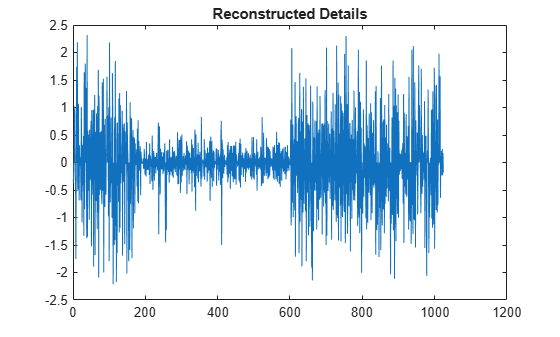wvarchg

Find variance change points

Description

example

[chgpts,kopt,est] = wvarchg(Y) computes estimated variation change points for the signal Y for six change points, where the minimum delay between two change points is 10.

[___] = wvarchg(Y,K) computes estimated variation change points for j change points, where j = 0, 1, 2, …, K, and the minimum delay between two change points is 10.

[___] = wvarchg(Y,K,D) computes estimated variation change points where the minimum delay between two change points is D.

• wvarchg(Y,6,10) is equivalent to wvarchg(Y).

• wvarchg(Y,K,10) is equivalent to wvarchg(Y,K).

Examples

collapse all

For reproducibility, set the random seed to the default value. Load the blocks wavelet test signal. Add white noise with two variance change points located at indices 180 and 600. Plot the noise and the noisy signal.

rng default
x = wnoise(1,10);
cp1 = 180;
cp2 = 600;
bb = 1.5*randn(1,length(x));
seg1 = bb(1:cp1);
seg2 = bb(cp1+1:cp2)/4;
seg3 = bb(cp2+1:end);
wn = [seg1 seg2 seg3];
x = x+wn;
subplot(2,1,1)
plot(wn)
title('Noise')
subplot(2,1,2)
plot(x)
title('Noisy Signal')Use the db3 wavelet and do a level-1 wavelet decomposition of the signal. Reconstruct the detail coefficients. Replace the top 2% of values with the mean value of the wavelet coefficients to remove most of the signal. Plot the values.

wname = 'db3';
lev = 1;
[c,l] = wavedec(x,lev,wname);
det = wrcoef('d',c,l,wname,1);
y = sort(abs(det));
v2p100 = y(fix(length(y)*0.98));
ind = find(abs(det)>v2p100);
det(ind) = mean(det);
figure
plot(det)
title('Reconstructed Details')Estimate the variance change points using the wavelet coefficients.

[pts_Opt,kopt,t_est] = wvarchg(det,5);
fprintf('The estimated change points are %d and %d.',pts_Opt)
The estimated change points are 181 and 601.

Input Arguments

collapse all

Input signal, specified as a real-valued vector. The input signal Y should have zero mean.

Data Types: double

Number of change points, specified as an integer. K satisfies the inequalities 1 < K ≪ length(Y).

Data Types: double

Number of change points, specified as an integer. D satisfies the inequalities 1 ≤ D ≪ length(Y).

Data Types: double

Output Arguments

collapse all

Estimated variance change points, returned as a vector. chgpts is the empty vector [] when no change points are found.

Proposed number of change points, returned as a nonnegative integer in the interval [0, k].

Instants of the variation change points, returned as a real-valued matrix. For 1 ≤ k ≤ K, est(k+1,1:k) contains the k instants of the variance change points. If kopt > 0, then chgpts = est(kopt+1,1:kopt), else chgpts = [].

 Lavielle, M. "Detection of multiple changes in a sequence of dependent variables." Stochastic Processes and their Applications. Vol. 83, Number 1, 1999, pp. 79–102.

Wavelet Toolbox DocumentationGet trial now